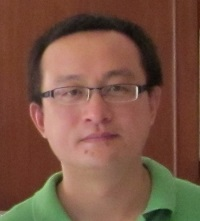Increasing Stability in the Inverse Source Problem with Attenuation and Many Frequencies
Speaker
Prof. Shuai Lu
Fudan University
Abstract

We study the interior inverse source problem for the Helmholtz equation from boundary Cauchy data of multiple wave numbers. The main goal of this paper is to understand the dependence of increasing stability on the attenuation, both analytically and numerically. To implement it we use the Fourier transform with respect to the wave numbers, explicit bounds for analytic continuation, and observability bounds for the wave equation. In particular, by using Carleman estimates for the wave equation we trace the dependence of exact observability bounds on the constant damping. Numerical examples in 3 spatial dimension support the theoretical results. It is a joint work with Prof. Victor Isakov (Wichita State University).